Examples

Chapter 5 Class 12 Continuity and Differentiability
Serial order wise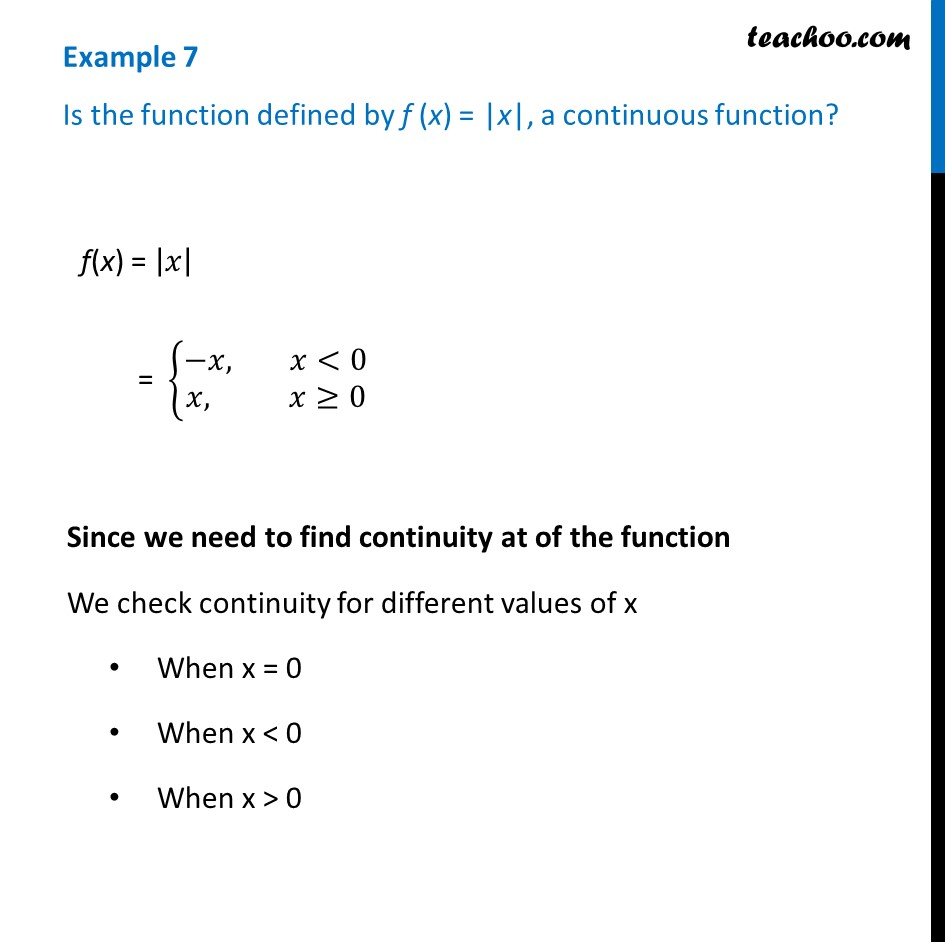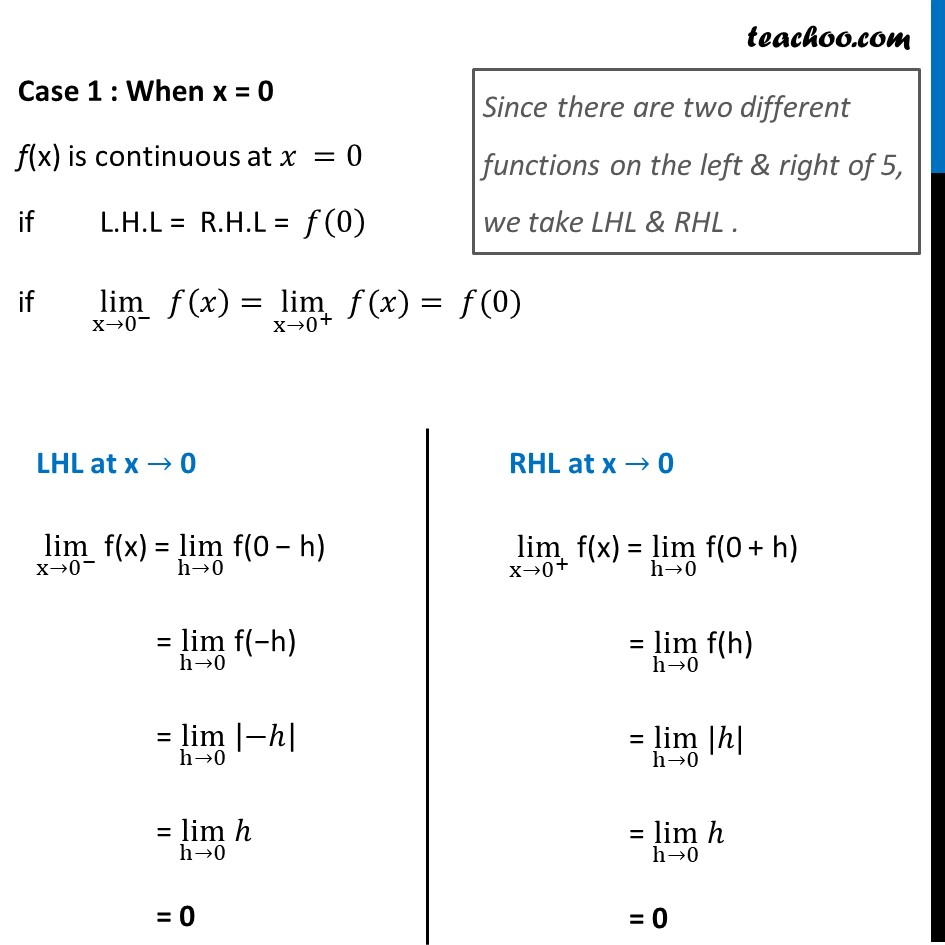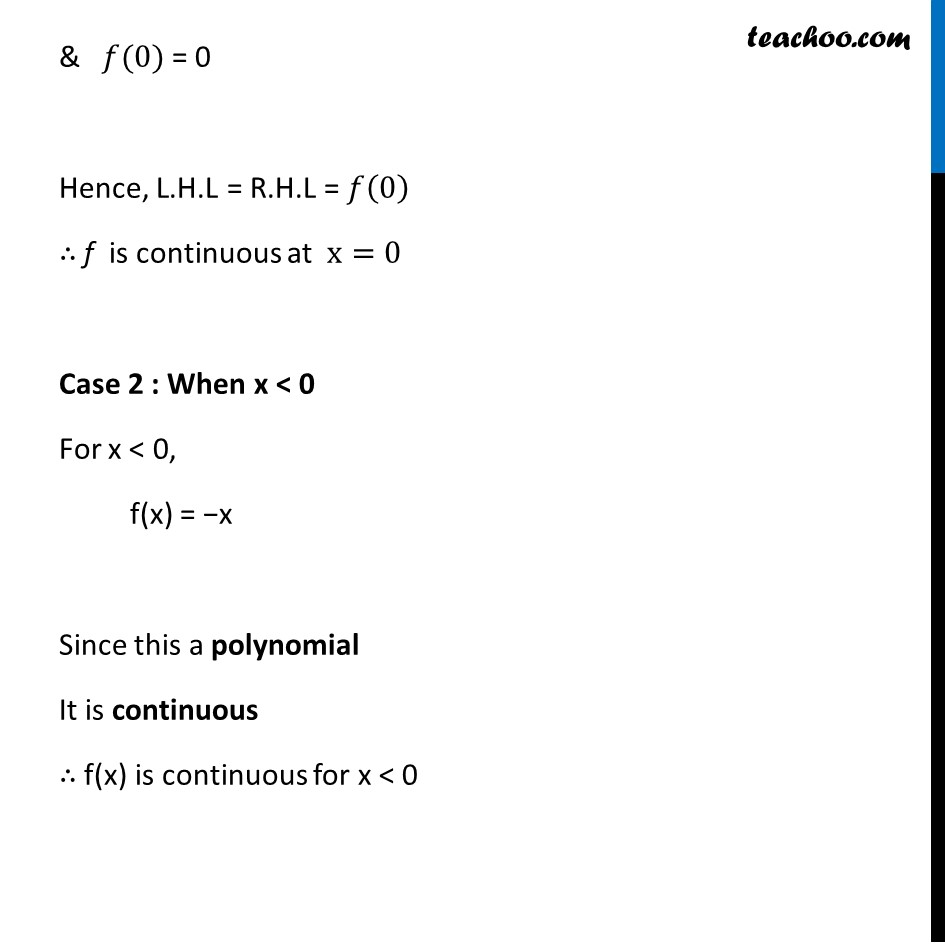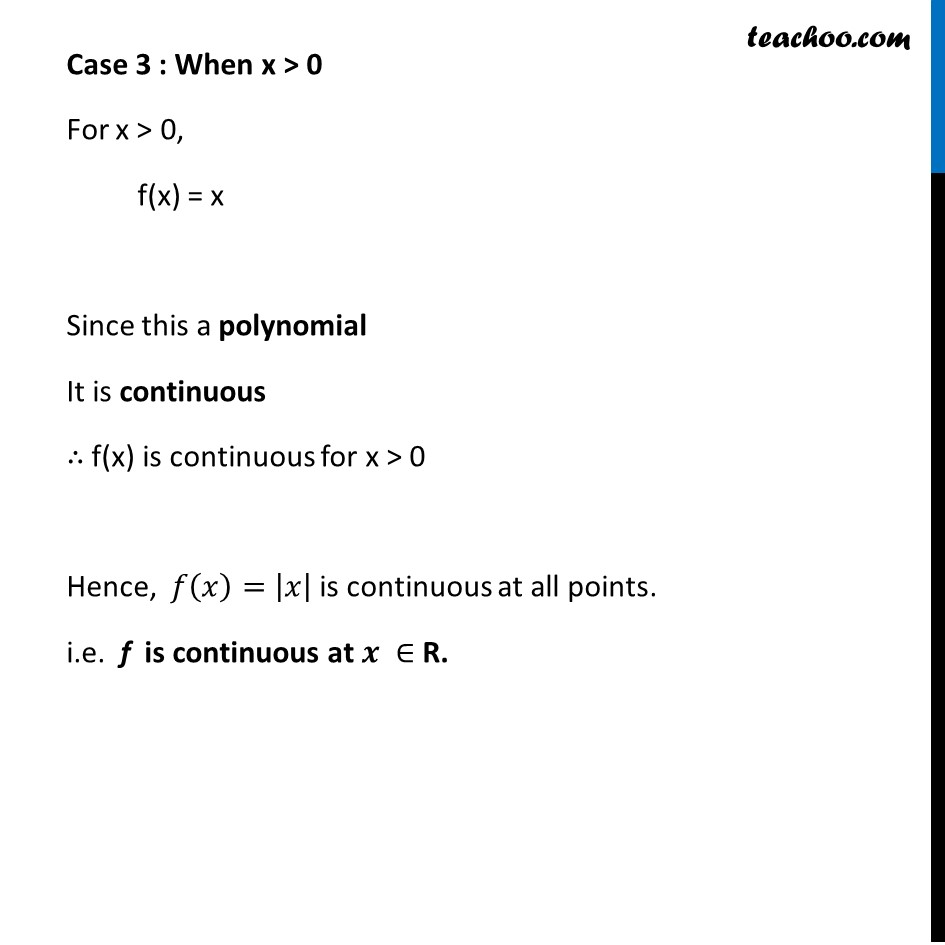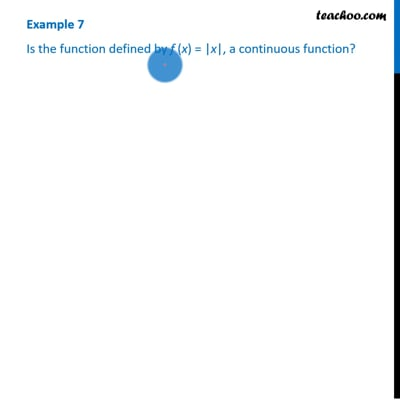This video is only available for Teachoo black users

Introducing your new favourite teacher - Teachoo Black, at only ₹83 per month

### Transcript

Example 7 Is the function defined by f (x) = |x|, a continuous function? f(x) = |𝑥| = {█(−𝑥, 𝑥<0@𝑥, 𝑥≥0)┤ Since we need to find continuity at of the function We check continuity for different values of x When x = 0 When x < 0 When x > 0 Case 1 : When x = 0 f(x) is continuous at 𝑥 =0 if L.H.L = R.H.L = 𝑓(0) if lim┬(x→0^− ) 𝑓(𝑥)=lim┬(x→0^+ ) " " 𝑓(𝑥)= 𝑓(0) Since there are two different functions on the left & right of 5, we take LHL & RHL . LHL at x → 0 lim┬(x→0^− ) f(x) = lim┬(h→0) f(0 − h) = lim┬(h→0) f(−h) = lim┬(h→0) |−ℎ| = lim┬(h→0) ℎ = 0 RHL at x → 0 lim┬(x→0^+ ) f(x) = lim┬(h→0) f(0 + h) = lim┬(h→0) f(h) = lim┬(h→0) |ℎ| = lim┬(h→0) ℎ = 0 & 𝑓(0) = 0 Hence, L.H.L = R.H.L = 𝑓(0) ∴ f is continuous at x=0 Case 2 : When x < 0 For x < 0, f(x) = −x Since this a polynomial It is continuous ∴ f(x) is continuous for x < 0 Case 3 : When x > 0 For x > 0, f(x) = x Since this a polynomial It is continuous ∴ f(x) is continuous for x > 0 Hence, 𝑓(𝑥)= |𝑥| is continuous at all points. i.e. f is continuous at 𝒙 ∈ R.